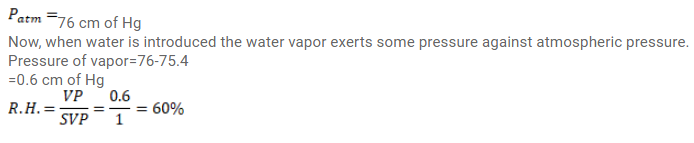# Use R=8.3 J/mol-K wherever required. A barometer tube is 80 cm long (above mercury reservoir).Question:

Use $\mathrm{R}=8.3 \mathrm{~J} / \mathrm{mol}-\mathrm{K}$ wherever required.

A barometer tube is $80 \mathrm{~cm}$ long (above mercury reservoir). It reads $76 \mathrm{~cm}$ on a particular day. A small amount of water is introduced in the tube and the reading drops to $75.4 \mathrm{~cm}$. Find the relative humidity in the space above the mercury column if the saturation vapor pressure at the room temperature is $1.0 \mathrm{~cm}$.

Solution: